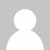Today’s challenge was certainly a tricky one, with the brute force solution effectively ruled out due to how long it would take. I did just about manage to get my 2 goal stars though – find out how by watching the video:

I actually tackled this in F# first. My solution to part a worked fine, but my algorithms for part b were too slow to solve the real input, leaving me looking elsewhere for inspiration. With limited time available I decided against implementing my own A* solution and borrow a trick from someone else. Judging by the subreddit, it seems many people found shortcuts or interesting properties of the input data allowing the search space to be reduced.

``````let mutate (sq:string) (replacements:(string*string) list) = seq {
for pos in [0 .. sq.Length - 1] do
for (a,b) in replacements do
if sq.Substring(pos).StartsWith(a) then
yield sq.Substring(0,pos) + b + sq.Substring(pos+a.Length)
}

let problemData = "day19.txt" |> File.ReadAllLines

let replacements =
problemData
|> Seq.map (fun s -> s.Split(' '))
|> Seq.filter (fun a -> a.Length = 3)
|> Seq.map (fun [|a;b;c|] -> (a,c))
|> Seq.toList

let medicineMolecule = problemData.[problemData.Length - 1]

mutate medicineMolecule replacements |> Seq.distinct |> Seq.length |> printfn "a: %d" // 509

let rand = new System.Random()

let swap (a: _[]) x y =
let tmp = a.[x]
a.[x] <- a.[y]
a.[y] <- tmp

// shuffle an array (in-place)
let shuffle a =
Array.iteri (fun i _ -> swap a i (rand.Next(i, Array.length a))) a

// What-a-baller algorithm
let search mol (reps:(string*string)[]) =
let mutable target = mol
let mutable mutations = 0

while not (target = "e") do
let mutable tmp = target
for a, b in reps do
let index = target.IndexOf(b)
if index >= 0 then
target <- target.Substring(0, index) + a + target.Substring(index + b.Length)
mutations <- mutations + 1

if tmp = target then
target <- mol
mutations <- 0
shuffle reps

mutations

search medicineMolecule (replacements |> List.toArray) |> printfn "b: %d" // 195

// note the search algorithm is not guaranteed to pick out the shortest solution:
search "XXXX" ([("y","XX");("e","yy");("e","XXXX")] |> List.toArray) |> printfn "broken: %d"
search "XXXX" ([("y","XX");("e","yy");("e","XXXX")] |> List.rev |> List.toArray) |> printfn "broken: %d"
``````

And here’s a C# version, taking pretty much the same approach:

``````IEnumerable<string> Mutate(string sq, IEnumerable<string[]> replacements)
{
return from pos in Enumerable.Range(0, sq.Length)
from rep in replacements
let a = rep
let b = rep
where sq.Substring(pos).StartsWith(a)
select sq.Substring(0,pos) + b + sq.Substring(pos+a.Length);
}

public static IEnumerable<T> Shuffle<T>(IEnumerable<T> source)
{
Random rnd = new Random();
return source.OrderBy<T, int>((item) => rnd.Next());
}

public int Search (string molecule, IEnumerable<string[]> replacements)
{
var target = molecule;
var mutations = 0;

while (target != "e")
{
var tmp = target;
foreach (var rep in replacements)
{
var a = rep;
var b = rep;
var index = target.IndexOf(b);
if (index >= 0)
{
target = target.Substring(0, index) + a + target.Substring(index + b.Length);
mutations++;
}
}

if (tmp == target)
{
target = molecule;
mutations = 0;
replacements = Shuffle(replacements).ToList();
}
}
return mutations;
}

void Main()
{
var replacements = problemData
.Select(s => s.Split(' '))
.Where(a => a.Length == 3)
.Select(a => new[] { a, a })
.ToList();

var medicineMolecule = problemData[problemData.Length - 1];

Mutate(medicineMolecule,replacements)
.Distinct()
.Count()
.Dump("a"); // 509

Search(medicineMolecule, replacements)
.Dump("b"); // 195
}
``````
Want to learn more about LINQ? Be sure to check out my Pluralsight course LINQ Best Practices.